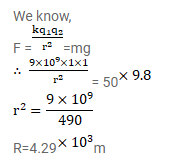# At what separation should two equal charges,Question:

At what separation should two equal charges, $1.0 \mathrm{C}$ each, be placed so that the force between them equals the weight of a $50 \mathrm{~kg}$ person?

Solution: